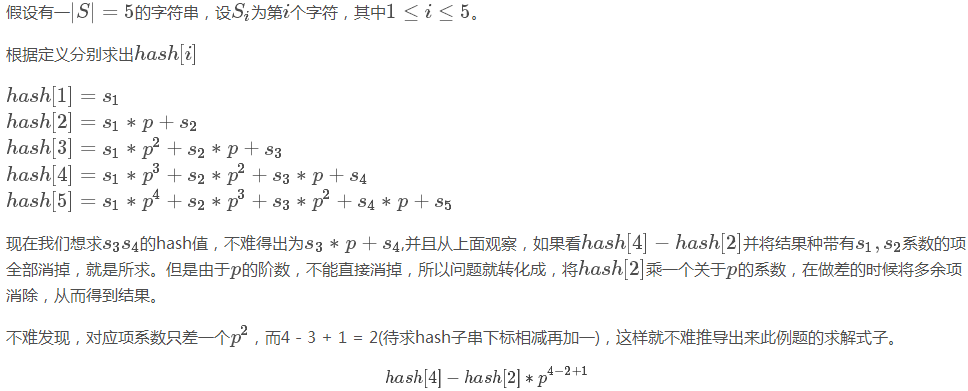## Pre-section

for (int i = 1; i <= n; i++) scanf("%d", &a), pos[a] = i;
for (int d = 1; d <= n / 2; d++)
for (int j = 1; j <= n - d - d; j++)
if ((pos[j] < pos[j + d] and pos[j + d] < pos[j + d + d]) or
(pos[j] > pos[j + d] and pos[j + d] > pos[j + d + d]))
{puts("Y"); break;}


BZOJ 上虽然数据范围相同但是跑不过去
CF 的 $3e5$的数据更是稳稳的过不去

$c$的值也是固定的

BZOJ 上还是过不了CF452F 改大一下数组去掉多组数据就可以了

#include <iostream>
#include <cstdio>
#include <cstring>
#include <cstdlib>
#include <complex>
#include <algorithm>
#include <climits>
#include <queue>
#include <map>
#include <set>
#include <vector>
#include <iomanip>
#define A 40010
#define B 2010

using namespace std;
typedef long long ll;
const int mod = 1e9 + 7;
struct node {
int l, r; ll w;
}tree[A];
int T, n, a[A >> 2]; ll p[A >> 2];
void build(int k, int l, int r, int f) {
tree[k][f].l = l; tree[k][f].r = r;
if (l == r) {
tree[k][f].w = 0;
return;
}
int m = (l + r) >> 1;
build(k << 1, l, m, f);
build(k << 1 | 1, m + 1, r, f);
}
void up(int k, int f) {
tree[k][f].w = tree[k << 1][f].w + tree[k << 1 | 1][f].w;
}
void change(int k, int pos, ll val, int f) {
if (tree[k][f].l == tree[k][f].r) {
tree[k][f].w = val;
return;
}
int m = (tree[k][f].l + tree[k][f].r) >> 1;
if (pos <= m) change(k << 1, pos, val, f);
else change(k << 1 | 1, pos, val, f);
up(k, f);
}
ll ask(int k, int l, int r, int f) {
if (tree[k][f].l >= l and tree[k][f].r <= r) return tree[k][f].w;
int m = (tree[k][f].l + tree[k][f].r) >> 1; ll ans = 0;
if (l <= m) ans = (ans + ask(k << 1, l, r, f)) % mod;
if (r > m) ans = (ans + ask(k << 1 | 1, l, r, f)) % mod;
return ans;
}

int main(int argc, char const *argv[]) {
p = 1; for (int i = 2; i <= 10000; i++) p[i] = (p[i - 1] * 123) % mod;
cin >> T;
while (T--) {
memset(tree, 0, sizeof tree);
scanf("%d", &n); build(1, 1, n, 0); build(1, 1, n, 1);
for (int i = 1; i <= n; i++) scanf("%d", &a[i]);
for (int i = 1; i <= n; i++) {
int len = min(a[i] - 1, n - a[i]); //回文半径不能越界

int s1 = a[i] - len, t1 = a[i]; //正向区间
ll ha1 = ask(1, s1, t1, 0); //正向哈希值

int s2 = n - a[i] - len + 1, t2 = n - a[i] + 1; //反向区间
ll ha2 = ask(1, s2, t2, 1); //反向哈希值

if (s2 > s1) ha1 = (ha1 * p[s2 - s1 + 1]) % mod; //对齐进制
else ha2 = (ha2 * p[s1 - s2 + 1]) % mod;
if (ha1 != ha2) {
puts("Y");
goto portal;
}
change(1, a[i], p[a[i]], 0); //分别修改两棵树
change(1, n + 1 - a[i], p[n - a[i] + 1], 1);
}
puts("N");
portal:;
}
return 0;
}


#### 良月澪二

EU gosto de música

### 1 条评论STO